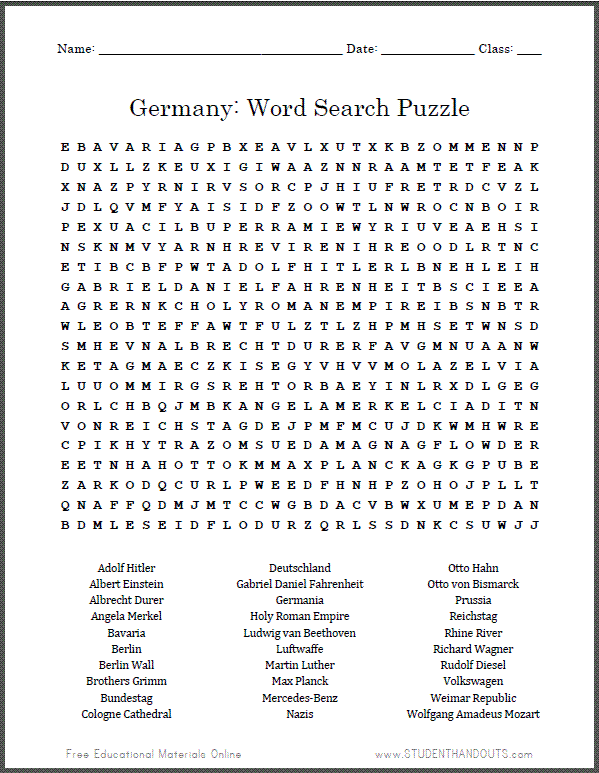﻿
Germany Word Search Puzzle | Student Handouts
 Share the learning joy!
 Germany Word Search PuzzleTERMS: Adolf Hitler, Albert Einstein, Albrecht Durer, Angela Merkel, Bavaria, Berlin, Berlin Wall, Brothers Grimm, Bundestag, Cologne Cathedral, Deutschland, Gabriel Daniel Fahrenheit, Germania, Holy Roman Empire, Ludwig van Beethoven, Luftwaffe, Martin Luther, Max Planck, Mercedes-Benz, Nazis, Otto Hahn, Otto von Bismarck, Prussia, Reichstag, Rhine River, Richard Wagner, Rudolf Diesel, Volkswagen, Weimar Republic, Wolfgang Amadeus Mozart.
 Click here to print. Click here for more of our free educational materials related to Germany.
 + B A V A R I A G + + + + A + L X + + + + + + O M + + N N + + U + + + + + E + + + + I + A + + N + + + + + T E + + E A + + N + + + + R + + + + S + R + + + + I U + + + T R D + V Z + + D + + + M + + + + S + D + + + + + + L N + + O C N B O I R + E + + A C I L B U P E R R A M I E W + R I + V E A E H S I N S + N + + + + R + H R E V I R E N I H R E O O D L R T N C E T I + + + + P + T A D O L F H I T L E R + B N E H L E I H G A B R I E L D A N I E L F A H R E N H E I T B S C I E E A A G R + + + + C H O L Y R O M A N E M P I R E I B S N B T R W + E + + + E F F A W T F U L + + + + + + + + S E T W N S D S + H + + N A L B R E C H T D U R E R + + + + M N U A A N W K E T + G + + + + + + + + + + + + + + + + + + A Z E L V I A L U U O M M I R G S R E H T O R B + + + + + + R + D L G E G O R L + + + + + + + + A N G E L A M E R K E L C + + + I T N V O N R E I C H S T A G + + + + + + + + + + + K + + + W R E C P I + + + T R A Z O M S U E D A M A G N A G F L O W D E R + E T N H A H O T T O + + M A X P L A N C K + + + + + U B + + A R + + + + + + + + + + + + + + + + + + + + + + + + L L + + N A + + + + + + + + + + + + + + + + + + + + + + + + + A + + + M L E S E I D F L O D U R + + + + + + + + + + + + + + +